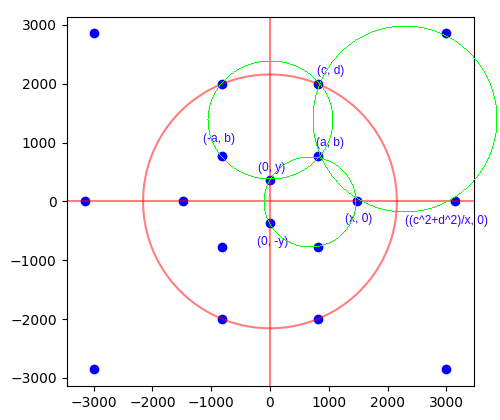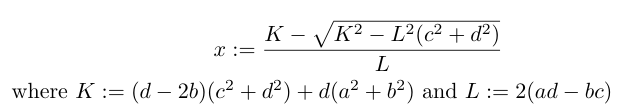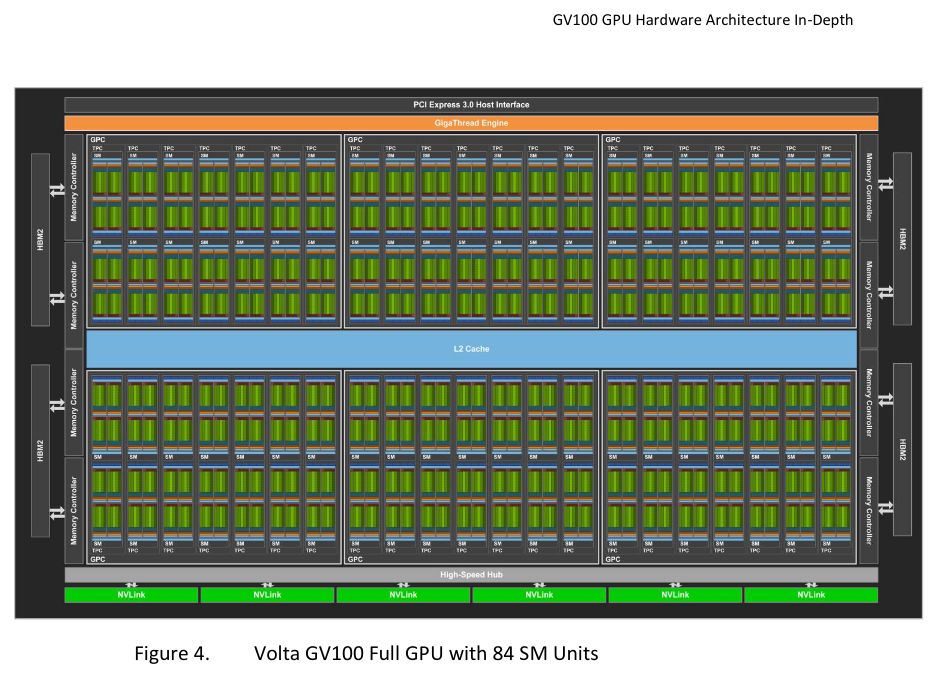# Another two rational dodecahedra

Since finding one rational dodecahedron inscribed in the unit sphere, I decided to port the search program to CUDA so that it can run on a GPU and thereby search a larger space in a reasonable amount of time. Firstly, let us recall our search methodology:We exhaustively look for all small positive integer points (a, b) and (c, d) satisfying b < d and ad > bc. The first of these inequalities ensures that (c, d) is further up the upper-left circle than (a, b); the second inequality ensures that the line through the origin and (a, b) intersects the upper-right circle at two distinct points.

For each such candidate pair of points, we analytically compute x and y, checking that they are rational. If so, we check that the four indicated points on the lower circle are indeed concyclic by performing a final determinant check.

This is embarrassingly parallel, so well-suited for a GPU. We launch one thread for each pair of 1 ≤ a, dN, where N = 10000, so a total of 10^8 threads are launched. Each thread performs two nested loops: the outer loop searches each value of b up to (but excluding) d; the inner loop starts with c = 1 and increments it until the inequality ad > bc is violated or c exceeds the bound N.

### Dealing with integer overflow

One rather annoying bugbear for searching a larger space was that of integer overflow. In the process of computing x, we need to take the square-root of a degree-6 polynomial in the four integer parameters a, b, c, d and check that this square-root is an integer*:*since the expression for x is rational if and only if$\sqrt{K^2 - L^2(c^2 + d^2)}$ is an integer.

When the integer parameters grow beyond 1000, this degree-6 polynomial can easily overflow the maximum representable 64-bit integer. To circumvent this problem, we take the following ugly approach:

• Compute$K^2 - L^2(c^2 + d^2)$ in both double-precision floating-point arithmetic (which is approximate) and in 64-bit integer arithmetic (which is exact, but reduced modulo 2^64).
• ‘Repair’ any loss of relative accuracy (caused by the subtraction) in the double-precision approximation by rounding to the nearest multiple of 2^64 and adding the exact result reduced into the interval [-2^63, 2^63 – 1].
• Compute the double-precision square-root. This will have comparable relative error, and therefore small absolute error (i.e. less than 1).
• Cast the square-root back to a 64-bit integer (which won’t overflow).
• Check this integer and nearby values to see whether they square (modulo 2^64) to the desired result.

Source code: here.

The analogous computation for y involves polynomials of degree at most 4, so we can safely search all a, b, c, d <= 10000 without overflow. The final determinant check is just the evaluation of a polynomial, so we can use arithmetic modulo 2^64 and not worry about integer overflow until later.

### Hardware

I opted to use an NVIDIA Volta V100 because it’s the most powerful GPU to which I currently have access (via Amazon Web Services). The V100 is huge, consisting of 80 streaming multiprocessors, each of which is capable of simultaneously issuing 4 instructions per cycle, where each instruction is vectorised over 32 ‘threads’. (For this reason, the GPU is sometimes advertised as having 80 × 4 × 32 = 5120 ‘CUDA cores’, but these are not comparable with CPU cores; a modern CPU core with vectorisation capabilities and multithreading is more akin to an entire streaming multiprocessor.)Schematic of the Volta V100 from the whitepaper. Only 80 of the 84 streaming multiprocessors are actually ‘activated’; this means that the fabrication of the chip is allowed to contain up to 4 manufacturing defects without affecting the validity of the resulting GPU.

Each of the 80 streaming multiprocessors also has 8 ‘tensor cores’, which perform reduced-precision matrix multiplications useful for neural networks. These tensor cores were unused by this search program, as the nature of this particular problem requires high-precision 64-bit integer and double-precision floating-point computations.

Compiling the program with the switch -Xptxas=-v shows that there is no expensive register spillage or other performance problems:

$nvcc -O3 -Xptxas=-v -lineinfo cudodeca.cu -o cudodeca ptxas info : 56 bytes gmem ptxas info : Compiling entry function '_Z12dodecakernelv' for 'sm_52' ptxas info : Function properties for _Z12dodecakernelv 64 bytes stack frame, 0 bytes spill stores, 0 bytes spill loads ptxas info : Used 47 registers, 320 bytes cmem, 24 bytes cmem Sixteen hours of continual uninterrupted runtime on the V100 (costing a total of about fifty US dollars) were sufficient to exhaust this space and show that there exist at most 3 primitive solutions within these bounds (full output here). The second and third solutions are much larger than the first: Solution 1: a=22, b=21, c=22, d=54, x=40, y=10 Solution 2: a=1680, b=1474, c=2023, d=2601, x=2890, y=578 Solution 3: a=2860, b=3915, c=3297, d=6594, x=7065, y=2355  The reason for saying ‘at most 3 primitive solutions’ is that the final determinant check is performed modulo 2^64, so false positives can slip through. To be absolutely sure of these results, we need an additional manual verification step using unbounded (‘bigint’) integer arithmetic. Unbounded integers are natively provided in languages such as Python, Haskell, and Wolfram Mathematica; we use the latter for convenience. ### Verification We can check the three determinants for the putative solution (1680, 1474, 2023, 2601, 2890, 578)… $ wolfram12.0.0
Mathematica 12.0.0 Kernel for Linux x86 (64-bit)

In:= f = Function[{x, y}, {1, x, y, x^2 + y^2}]

Out= Function[{x, y}, {1, x, y, x^2 + y^2}]

In:= Det[{f[a, b], f[x, 0], f[0, y], f[0, -y]}] /. {a -> 1680, b -> 1474, c -> 2023, d -> 2601, x -> 2890, y -> 578}

Out= 0

In:= Det[{f[(c^2+d^2)/x, 0], f[c, d], f[a, b], f[x, 0]}] /. {a -> 1680, b -> 1474, c -> 2023, d -> 2601, x -> 2890, y -> 578}

Out= 0

In:= Det[{f[c, d], f[a, b], f[-a, b], f[0, y]}] /. {a -> 1680, b -> 1474, c -> 2023, d -> 2601, x -> 2890, y -> 578}

Out= 0

…and likewise for the putative solution (2860, 3915, 3297, 6594, 7065, 2355)…

In:= Det[{f[a, b], f[x, 0], f[0, y], f[0, -y]}] /. {a -> 2860, b -> 3915, c -> 3297, d -> 6594, x -> 7065, y -> 2355}

Out= 0

In:= Det[{f[c, d], f[a, b], f[-a, b], f[0, y]}] /. {a -> 2860, b -> 3915, c -> 3297, d -> 6594, x -> 7065, y -> 2355}

Out= 0

In:= Det[{f[(c^2+d^2)/x, 0], f[c, d], f[a, b], f[x, 0]}] /. {a -> 2860, b -> 3915, c -> 3297, d -> 6594, x -> 7065, y -> 2355}

Out= 0

…confirming that these are indeed valid primitive solutions.

This entry was posted in Uncategorized. Bookmark the permalink.

### 4 Responses to Another two rational dodecahedra

1.Anonymous says:

It turns out that x and y can be written as a rational expression in terms of a, b, c, d (albeit with unwieldy and extremely high degree terms!) by eliminating the terms from the three equations. You then end up with a single homogeneous degree 30 equation in a, b, c, and d. Unfortunately, the algebra system I am using is currently crashing trying to figure out what that equation is. I’m hoping that factors drop out that reduce the degree and allow for an easier solution. Hopefully this will allow us to find an infinite family of solutions.

•Anonymous says:

Actually, more like degree 22 after removing easy factors. But still, it’s a ridiculous equation.

•apgoucher says:

Interesting! Did you make any further progress on simplifying the equation without causing the computer algebra system to crash?